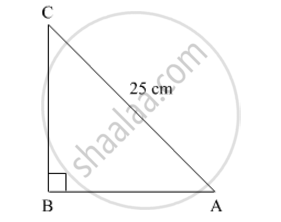# The perimeter of a right triangle is 60 cm. Its hypotenuse is 25 cm. Find the area of the triangle. - Mathematics

The perimeter of a right triangle is 60 cm. Its hypotenuse is 25 cm. Find the area of the triangle.

#### SolutionLet ABC be a right-angled triangle.

Since the perimeter of the right triangle is 60 cm,

AB + BC +CA = 60 cm

⇒ AB + BC + 25 = 60

⇒ AB + BC = 35 cm          .....(1)

In ∆ABC,

AB2 + BC2 = CA2

⇒ (AB + BC)2 − 2(AB)(BC) = (25)2

⇒ (35)2 − 2(AB)(BC) = (25)2                                     [From (1)]

⇒ (35 − 25)(35 + 25) = 2(AB)(BC)'

⇒ (AB)(BC) = 300

Now,

Area of ABC1/2×AB×BC=1/2×300=150 cm2

Hence, the area of the triangle is 150 cm2.

Concept: Area of a Triangle
Is there an error in this question or solution?
2015-2016 (March) Delhi Set 2

Share